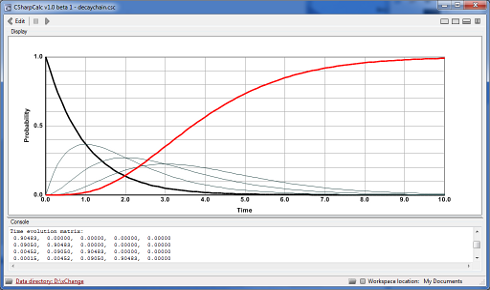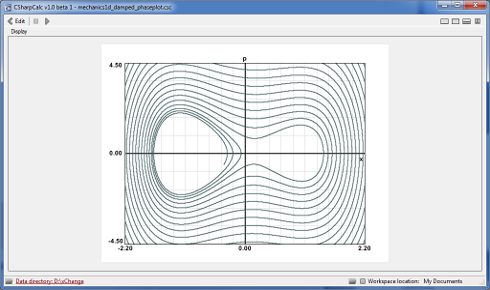# CSharpCalc Screenshots

This page gives a visual impression of some aspects of CSharpCalc. The software consists of two distinct parts, the code editor and the display. Both parts are integrated in one compact user interface.The CSharpCalc code editor is a modern text editor with syntax highlighting, dynamical page numbering and the ability to collapse and expand parts of the source code. Below the code editor you see a list of compiler messages.Formatted numerical output written to the CSharpCalc console. The software supports the full text formatting functionality comming with the C# language.Graphical output generated with CSharpCalc. This screenshot displays the magnetisation as function of the temperature as calculated in the mean-field Ising model for four different values of the external field h. The implicit equation of state, describing the magnetization as function of the temperature, is solved directly in the visualization script using Newton iterations.

# Miscellaneous visuals made with CSharpCalc

CSharpCalc can be used in various ways and in virtually all fields of science using mathematical calculations and models. Below, three salient examples. More visuals can be found in the showcase section.Population probability densities of a five-element decay chain as functions of time. The population probability of the unstable parent element is printed black, the stable element's population probability is printed in red.CSharpcalc visualizing a wave packet with a gaussian enveloping function. The envelope is drawn as thick black line. The actual amplitude of the wave packet is drawn with a thin slate line.Hodograph of a damped anharmonic oscillator. The Newtonian equations of motion were solved numerically for a fourth-order anharmonic oscillator potential with a linear friction force added.